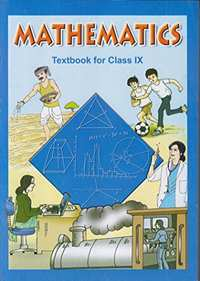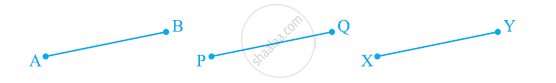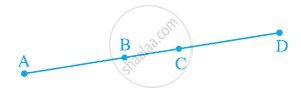# NCERT solutions for Class 9 Maths chapter 5 - Introduction to Euclid's Geometry [Latest edition]

#### Chapters## Solutions for Chapter 5: Introduction to Euclid's Geometry

Below listed, you can find solutions for Chapter 5 of CBSE NCERT for Class 9 Maths.

Exercise 5.1Exercise 5.2
Exercise 5.1 [Pages 85 - 86]

### NCERT solutions for Class 9 Maths Chapter 5 Introduction to Euclid's Geometry Exercise 5.1 [Pages 85 - 86]

#### Which of the following statements are true and which are false? Give reasons for your answers

Exercise 5.1 | Q 1. (i) | Page 85

Only one line can pass through a single point.

• True

• False

Exercise 5.1 | Q 1. (ii) | Page 85

There are an infinite number of lines which pass through two distinct points.

• True

• False

Exercise 5.1 | Q 1. (iii) | Page 85

A terminated line can be produced indefinitely on both the sides.

• True

• False

Exercise 5.1 | Q 1. (iv) | Page 85

If two circles are equal, then their radii are equal.

• True

• False

Exercise 5.1 | Q 1. (v) | Page 85

In the following figure, if AB = PQ and PQ = XY, then AB = XY• True

• False

Exercise 5.1 | Q 2.1 | Page 85

Give a definition for parallel lines. Are there other terms that need to be defined first? What are they, and how might you define them?

Exercise 5.1 | Q 2.2 | Page 85

Give a definition for perpendicular lines. Are there other terms that need to be defined first? What are they, and how might you define them?

Exercise 5.1 | Q 2.3 | Page 85

Give a definition for line segment. Are there other terms that need to be defined first? What are they, and how might you define them?

Exercise 5.1 | Q 2.4 | Page 85

Give a definition for radius of a circle. Are there other terms that need to be defined first? What are they, and how might you define them?

Exercise 5.1 | Q 2.5 | Page 85

Give a definition for square. Are there other terms that need to be defined first? What are they, and how might you define them?

Exercise 5.1 | Q 3 | Page 85

Consider two ‘postulates’ given below:-

(i) Given any two distinct points A and B, there exists a third point C which is in between A and B.

(ii) There exist at least three points that are not on the same line.

Do these postulates contain any undefined terms? Are these postulates consistent? Do they follow from Euclid’s postulates? Explain.

Exercise 5.1 | Q 4 | Page 86

If a point C lies between two points A and B such that AC = BC, then prove that Ac =  1/2AB. Explain by drawing the figure.

Exercise 5.1 | Q 5 | Page 86

If a point C lies between two points A and B such that AC = BC, point C is called a mid-point of line segment AB. Prove that every line segment has one and only one mid-point.

Exercise 5.1 | Q 6 | Page 86

In the following figure, if AC = BD, then prove that AB = CD.Exercise 5.1 | Q 7 | Page 86

Why is Axiom 5, in the list of Euclid’s axioms, considered a ‘universal truth’? (Note that the question is not about the fifth postulate.)

Exercise 5.2 [Page 88]

### NCERT solutions for Class 9 Maths Chapter 5 Introduction to Euclid's Geometry Exercise 5.2 [Page 88]

Exercise 5.2 | Q 1 | Page 88

How would you rewrite Euclid’s fifth postulate so that it would be easier to understand?

Exercise 5.2 | Q 2 | Page 88

Does Euclid’s fifth postulate imply the existence of parallel lines? Explain.

## Solutions for Chapter 5: Introduction to Euclid's Geometry

Exercise 5.1Exercise 5.2## NCERT solutions for Class 9 Maths chapter 5 - Introduction to Euclid's Geometry

Shaalaa.com has the CBSE Mathematics Class 9 Maths CBSE solutions in a manner that help students grasp basic concepts better and faster. The detailed, step-by-step solutions will help you understand the concepts better and clarify any confusion. NCERT solutions for Mathematics Class 9 Maths CBSE 5 (Introduction to Euclid's Geometry) include all questions with answers and detailed explanations. This will clear students' doubts about questions and improve their application skills while preparing for board exams.

Further, we at Shaalaa.com provide such solutions so students can prepare for written exams. NCERT textbook solutions can be a core help for self-study and provide excellent self-help guidance for students.

Concepts covered in Class 9 Maths chapter 5 Introduction to Euclid's Geometry are Concept for Euclid’S Geometry, Euclid’S Definitions, Axioms and Postulates, Equivalent Versions of Euclid’S Fifth Postulate.

Using NCERT Class 9 Maths solutions Introduction to Euclid's Geometry exercise by students is an easy way to prepare for the exams, as they involve solutions arranged chapter-wise and also page-wise. The questions involved in NCERT Solutions are essential questions that can be asked in the final exam. Maximum CBSE Class 9 Maths students prefer NCERT Textbook Solutions to score more in exams.

Get the free view of Chapter 5, Introduction to Euclid's Geometry Class 9 Maths additional questions for Mathematics Class 9 Maths CBSE, and you can use Shaalaa.com to keep it handy for your exam preparation.